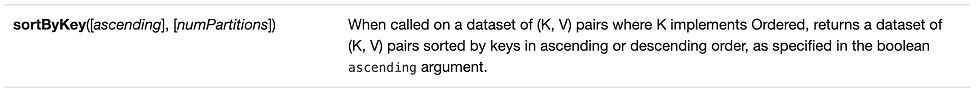top of page
BlogPageTop

# Spark Transformation example in Scala (Part 2)

Updated: Nov 18, 2022

In this post I will walk you through groupByKey, reduceByKey, aggregateByKey, sortByKey, join, cartesian, coalesce and repartition Spark transformations.

In previous blog we covered map, flatMap, mapPartitions, mapPartitionsWithIndex, filter, distinct, union, intersection and sample Spark transformations. I would encourage you all to go through these posts before this.

### groupByKey()As name says it groups the dataset (K, V) key-value pair based on Key and stores the value as Iterable, (K, V) => (K, Iterable(V)). It's very expensive operation and consumes lot of memory if dataset is huge. For example,

scala> val rdd = sc.parallelize(List("Hello Hello Spark Apache Hello Dataneb Dataneb Dataneb Spark"))

rdd: org.apache.spark.rdd.RDD[String] = ParallelCollectionRDD at parallelize at <console>:24

scala> rdd.collect

res3: Array[String] = Array(Hello Hello Spark Apache Hello Dataneb Dataneb Dataneb Spark)

// Splitting the array and creating (K, V) pair

scala> val keyValue = rdd.flatMap(words => words.split(" ")).map(x=>(x,1))

keyValue: org.apache.spark.rdd.RDD[(String, Int)] = MapPartitionsRDD at map at <console>:25

// Iterable[Int] Value "1" tells number of occurrences of Key

scala> keyValue.groupByKey.collect

res12: Array[(String, Iterable[Int])] = Array((Spark,CompactBuffer(1, 1)), (Dataneb,CompactBuffer(1, 1, 1)), (Hello,CompactBuffer(1, 1, 1)), (Apache,CompactBuffer(1)))

### reduceByKey()Operates on (K, V) pair dataset, but reduce func must be of type (V, V) => V. For example, if you want to reduce all the values to get the total number of occurrences.

scala> rdd

.flatMap(words => words.split(" "))

.map(x=>(x,1))

.reduceByKey((x, y)=>x+y)

.collect

res14: Array[(String, Int)] = Array((Spark,2), (Dataneb,3), (Hello,3), (Apache,1))

### aggregateByKey()It's similar to reduceByKey(), I hardly use this transformation because you can achieve the same with previous transformation. For example,

scala> rdd

.flatMap(words => words.split(" "))

.map(x=>(x,1))

.aggregateByKey(0)((x, y)=> x+y, (k, v)=> k+v )

.collect

res24: Array[(String, Int)] = Array((Spark,2), (Dataneb,3), (Hello,3), (Apache,1))

### sortByKey()Called upon key-value pair, returns sorted by keys. For example,

scala> rdd

.flatMap(words => words.split(" "))

.distinct

.map(x => (x,1))

.sortByKey() -- by default Ascending

.collect

res36: Array[(String, Int)] = Array((Apache,1), (Dataneb,1), (Hello,1), (Spark,1))

scala> rdd

.flatMap(words => words.split(" "))

.distinct

.map(x => (x,1))

.sortByKey(false) -- Ascending order (false)

.collect

res37: Array[(String, Int)] = Array((Spark,1), (Hello,1), (Dataneb,1), (Apache,1))

### join()It takes datasets of type key-value pair and works same like sql joins. For no match value will be None. For example,

scala> val rdd1 = sc.parallelize(List("Apple","Orange", "Banana", "Grapes", "Strawberry", "Papaya")).map(words => (words,1))

rdd1: org.apache.spark.rdd.RDD[(String, Int)] = MapPartitionsRDD at map at <console>:24

scala> val rdd2 = sc.parallelize(List("Apple", "Grapes", "Peach", "Fruits")).map(words => (words,1))

rdd2: org.apache.spark.rdd.RDD[(String, Int)] = MapPartitionsRDD at map at <console>:24

scala> rdd1.join(rdd2).collect

res40: Array[(String, (Int, Int))] = Array((Grapes,(1,1)), (Apple,(1,1)))

scala> rdd1.rightOuterJoin(rdd2).collect

res41: Array[(String, (Option[Int], Int))] = Array((Grapes,(Some(1),1)), (Peach,(None,1)), (Apple,(Some(1),1)), (Fruits,(None,1)))

scala> rdd1.leftOuterJoin(rdd2).collect

res43: Array[(String, (Int, Option[Int]))] = Array((Grapes,(1,Some(1))), (Banana,(1,None)), (Papaya,(1,None)), (Orange,(1,None)), (Apple,(1,Some(1))), (Strawberry,(1,None)))

scala> rdd1.fullOuterJoin(rdd2).collect

res44: Array[(String, (Option[Int], Option[Int]))] = Array((Grapes,(Some(1),Some(1))), (Peach,(None,Some(1))), (Banana,(Some(1),None)), (Papaya,(Some(1),None)), (Orange,(Some(1),None)), (Apple,(Some(1),Some(1))), (Fruits,(None,Some(1))), (Strawberry,(Some(1),None)))

### cartesian()Same like cartesian product, return all possible pairs of elements of dataset.

scala> val rdd1 = sc.parallelize(List("Apple","Orange", "Banana", "Grapes", "Strawberry", "Papaya"))

rdd1: org.apache.spark.rdd.RDD[String] = ParallelCollectionRDD at parallelize at <console>:24

scala> val rdd2 = sc.parallelize(List("Apple", "Grapes", "Peach", "Fruits"))

rdd2: org.apache.spark.rdd.RDD[String] = ParallelCollectionRDD at parallelize at <console>:24

scala> rdd1.cartesian(rdd2).collect

res46: Array[(String, String)] = Array((Apple,Apple), (Apple,Grapes), (Apple,Peach), (Apple,Fruits), (Orange,Apple), (Banana,Apple), (Orange,Grapes), (Banana,Grapes), (Orange,Peach), (Banana,Peach), (Orange,Fruits), (Banana,Fruits), (Grapes,Apple), (Grapes,Grapes), (Grapes,Peach), (Grapes,Fruits), (Strawberry,Apple), (Papaya,Apple), (Strawberry,Grapes), (Papaya,Grapes), (Strawberry,Peach), (Papaya,Peach), (Strawberry,Fruits), (Papaya,Fruits))

### coalesce()coalesce and repartition both shuffles the data to increase or decrease the partition, but repartition is more costlier operation as it re-shuffles all data and creates new partition. For example,

scala> val distData = sc.parallelize(1 to 16, 4)

distData: org.apache.spark.rdd.RDD[Int] = ParallelCollectionRDD at parallelize at <console>:24

// current partition size

scala> distData.partitions.size

res63: Int = 4

// checking data across each partition

scala> distData.mapPartitionsWithIndex((index, iter) => if (index == 0) iter else Iterator()).collect

res64: Array[Int] = Array(1, 2, 3, 4)

scala> distData.mapPartitionsWithIndex((index, iter) => if (index == 1) iter else Iterator()).collect

res65: Array[Int] = Array(5, 6, 7, 8)

scala> distData.mapPartitionsWithIndex((index, iter) => if (index == 2) iter else Iterator()).collect

res66: Array[Int] = Array(9, 10, 11, 12)

scala> distData.mapPartitionsWithIndex((index, iter) => if (index == 3) iter else Iterator()).collect

res67: Array[Int] = Array(13, 14, 15, 16)

// decreasing partitions to 2

scala> val coalData = distData.coalesce(2)

coalData: org.apache.spark.rdd.RDD[Int] = CoalescedRDD at coalesce at <console>:25

// see how shuffling occurred. Instead of moving all data it just moved 2 partitions.

scala> coalData.mapPartitionsWithIndex((index, iter) => if (index == 0) iter else Iterator()).collect

res68: Array[Int] = Array(1, 2, 3, 4, 5, 6, 7, 8)

scala> coalData.mapPartitionsWithIndex((index, iter) => if (index == 1) iter else Iterator()).collect

res69: Array[Int] = Array(9, 10, 11, 12, 13, 14, 15, 16)

### repartition()Notice how it re-shuffled everything to create new partitions as compared to previous RDDs - distData and coalData. Hence repartition is more costlier operation as compared to coalesce.

scala> val repartData = distData.repartition(2)

repartData: org.apache.spark.rdd.RDD[Int] = MapPartitionsRDD at repartition at <console>:25

// checking data across each partition

scala> repartData.mapPartitionsWithIndex((index, iter) => if (index == 0) iter else Iterator()).collect

res70: Array[Int] = Array(1, 3, 6, 8, 9, 11, 13, 15)

scala> repartData.mapPartitionsWithIndex((index, iter) => if (index == 1) iter else Iterator()).collect

res71: Array[Int] = Array(2, 4, 5, 7, 10, 12, 14, 16)

That's all folks. If you have any question please mention in comments section below. Thank you.

### Next: Spark RDD, Transformation and Actions

1. Apache Spark and Scala Installation

2. Getting Familiar with Scala IDE

3. Spark data structure basics

4. Spark Shell

5. Reading data files in Spark

6. Writing data files in Spark

7. Spark streaming